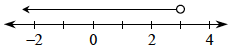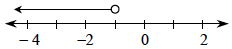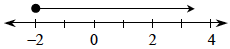### Home > CCAA > Chapter 7 Unit 6 > Lesson CC2: 7.1.5 > Problem7-60

7-60.

Write the inequality represented by each graph.

1.Since the arrow is pointing to the left, that means that all those values are less than the selected point.

Therefore, any value to the left of $3$ is less than $3$. Since the circle above the selected point is open, the point itself is not included and you need to use either $<$ or $>$.

$x<3$

1.See part (a).

1.Notice that this time the circle above the selected point is shaded in, so the point itself is included. That means you will use either $\le$ or $\ge$. Since the arrow is pointing to the right, that means that all those values are greater than the selected point.